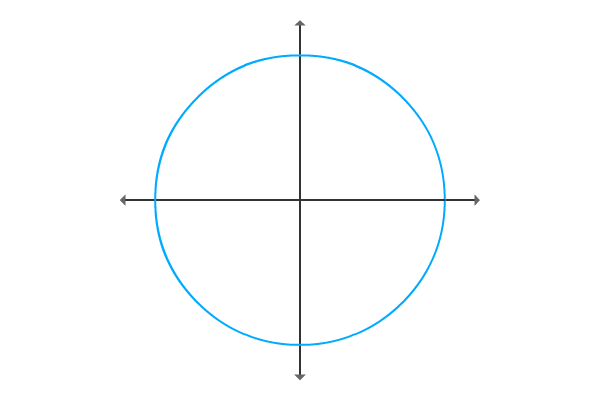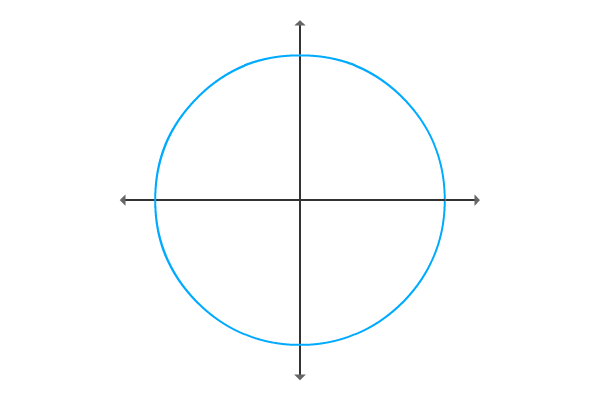Sin of negative angle

A mathematical relation of sine of negative angle with sine of positive angle is called sine of negative angle identity.

Formula

$\sin{(-\theta)} \,=\, -\sin{\theta}$

Proof

The mathematical relation between sine of angle and sine of negative angle can be derived in mathematical form in trigonometry by geometrical approach.

Construction of triangle with positive angle$\Delta QOP$ is a right angled triangle and it is constructed with an angle theta. Sine function depends on the lengths of the opposite side and hypotenuse. So, express sine of positive angle in terms of ratio of lengths of the respective sides.

$\sin{\theta} \,=\, \dfrac{PQ}{OP}$

The $\Delta QOP$ is in first quadrant. So, the lengths of opposite side and adjacent sides are positive. Take the lengths of them are $y$ and $x$ respectively.

$\implies$ $\sin{\theta} \,=\, \dfrac{y}{\sqrt{x^2+y^2}}$

Construction of triangle with negative angleNow, construct same triangle with opposite angle but the magnitude should be same. So, the angle of the $\Delta QOR$ is negative theta ($-\theta$).

Write trigonometric ratio sine in terms of ratio of the lengths of the sides.

$\sin{(-\theta)} \,=\, \dfrac{RQ}{OR}$

The length of the opposite side is negative due to construction of the triangle with negative angle. Therefore, the length of the opposite side will be $–y$ but the length of the adjacent side remains same geometrically.

$\implies$ $\sin{(-\theta)} \,=\, \dfrac{-y}{\sqrt{x^2+y^2}}$

Comparing sine functions

Compare equations of both sine of positive angle and sine of negative angle.

$\sin{\theta} \,=\, \dfrac{y}{\sqrt{x^2+y^2}}$

$\sin{(-\theta)} \,=\, -\dfrac{y}{\sqrt{x^2+y^2}}$

The two equations discloses that sine of negative angle is always equal to negative sine of angle.

$\sin{(-\theta)} \,=\, -(\sin{\theta})$

$\,\,\, \therefore \,\,\,\,\,\,$ $\sin{(-\theta)} \,=\, -\sin{\theta}$

It is called sine of negative angle identity and used as a formula in trigonometry mathematics.

Latest Math Problems
Email subscription
Math Doubts is a best place to learn mathematics and from basics to advanced scientific level for students, teachers and researchers. Know more